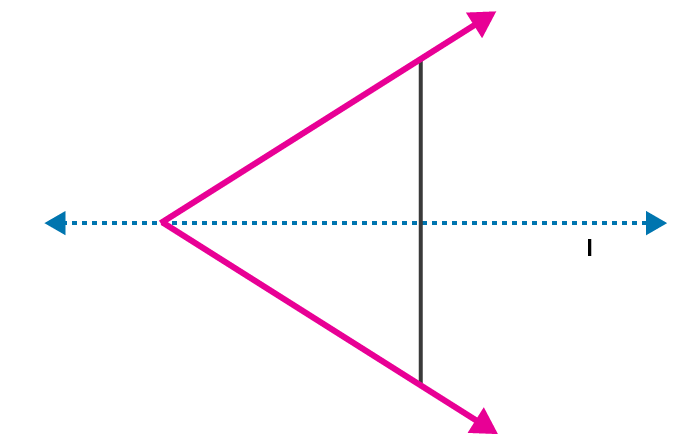# ML Aggarwal Solutions for Class 6 Maths Chapter 12 Symmetry

ML Aggarwal Solutions for Class 6 Maths Chapter 12 Symmetry explains all the fundamental concepts, with illustrations. Students who want to score high marks in the exams, are advised to revise these solutions on a daily basis. This also helps them to obtain an idea of questions which would appear in the final examination. To know more about the concepts covered here, students can refer to ML Aggarwal Solutions for Class 6 Maths Chapter 12 Symmetry PDF, from the links provided below

Chapter 12 mainly deals with the study of problems related to lines of Symmetry. These solutions designed in a simple language boost confidence in solving complex problems among students with ease. It helps them to improve their problem solving and analytical thinking abilities, which are important from the exam point of view.

## ML Aggarwal Solutions for Class 6 Maths Chapter 12 Symmetry Download PDF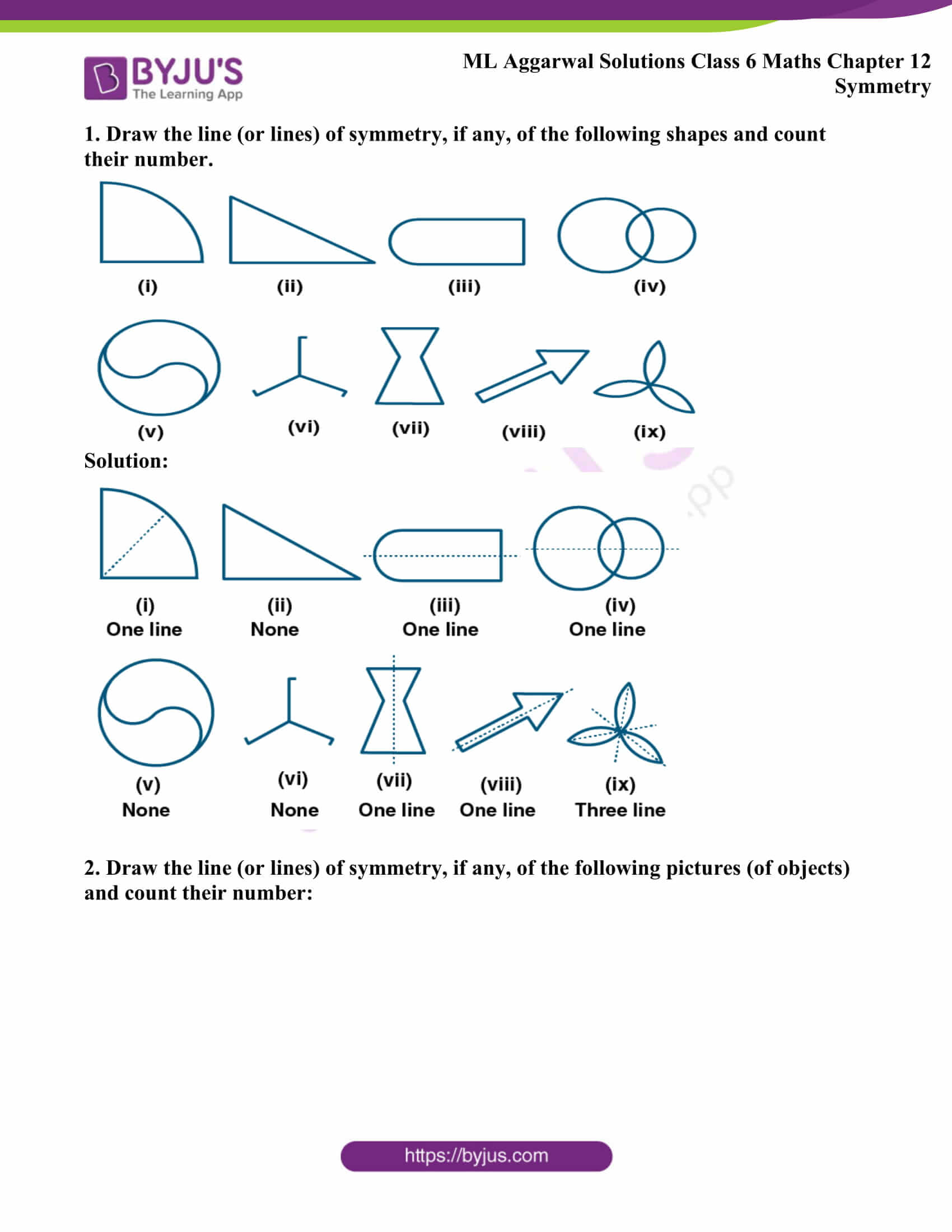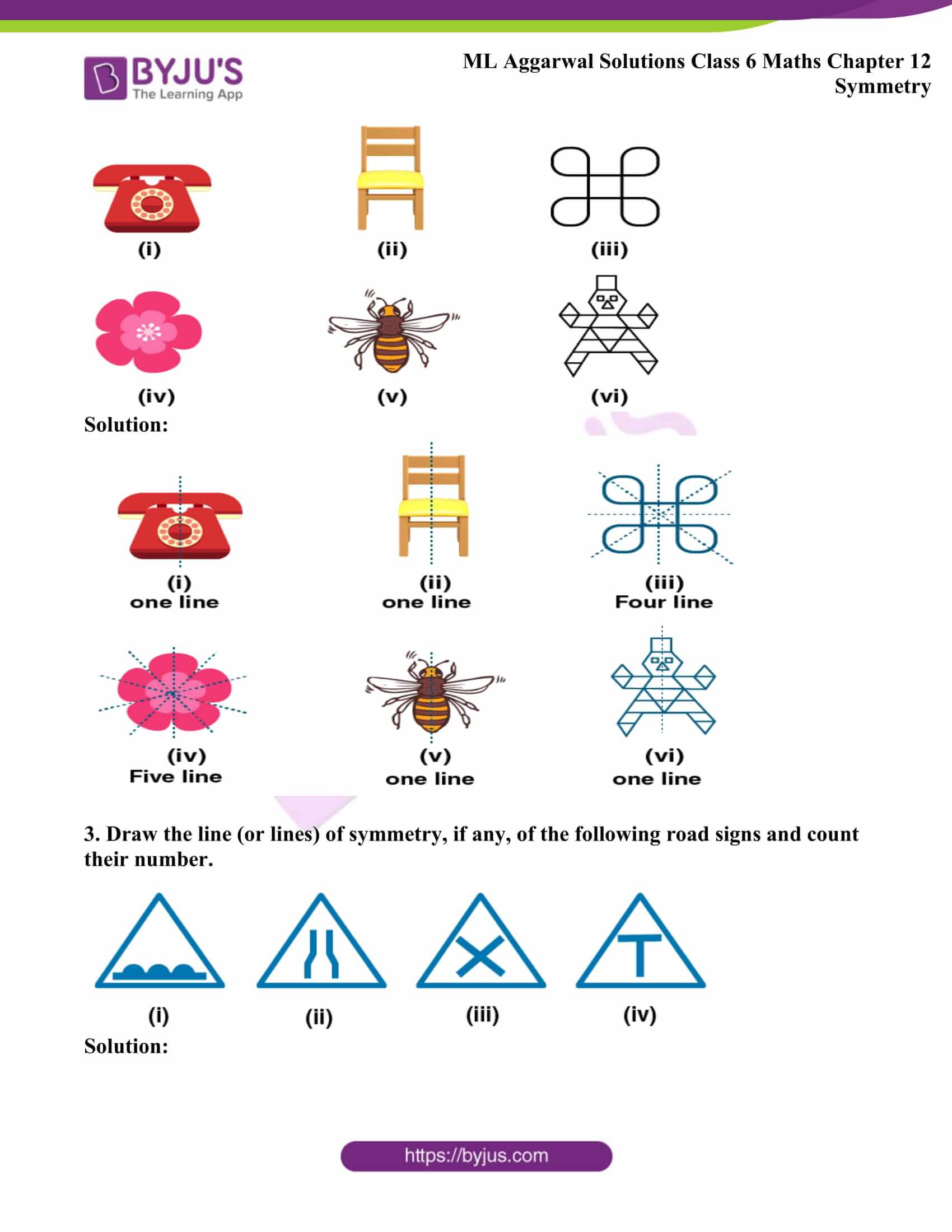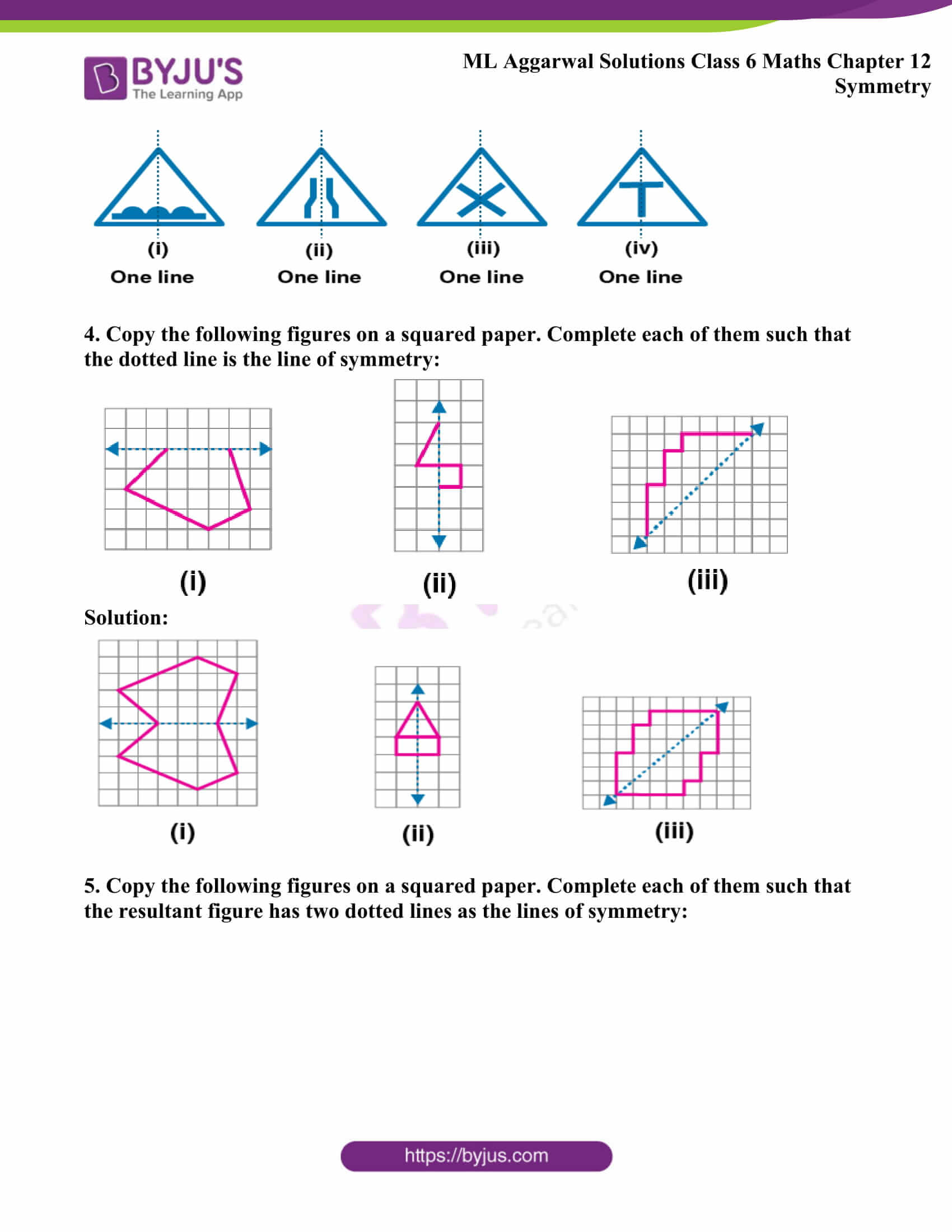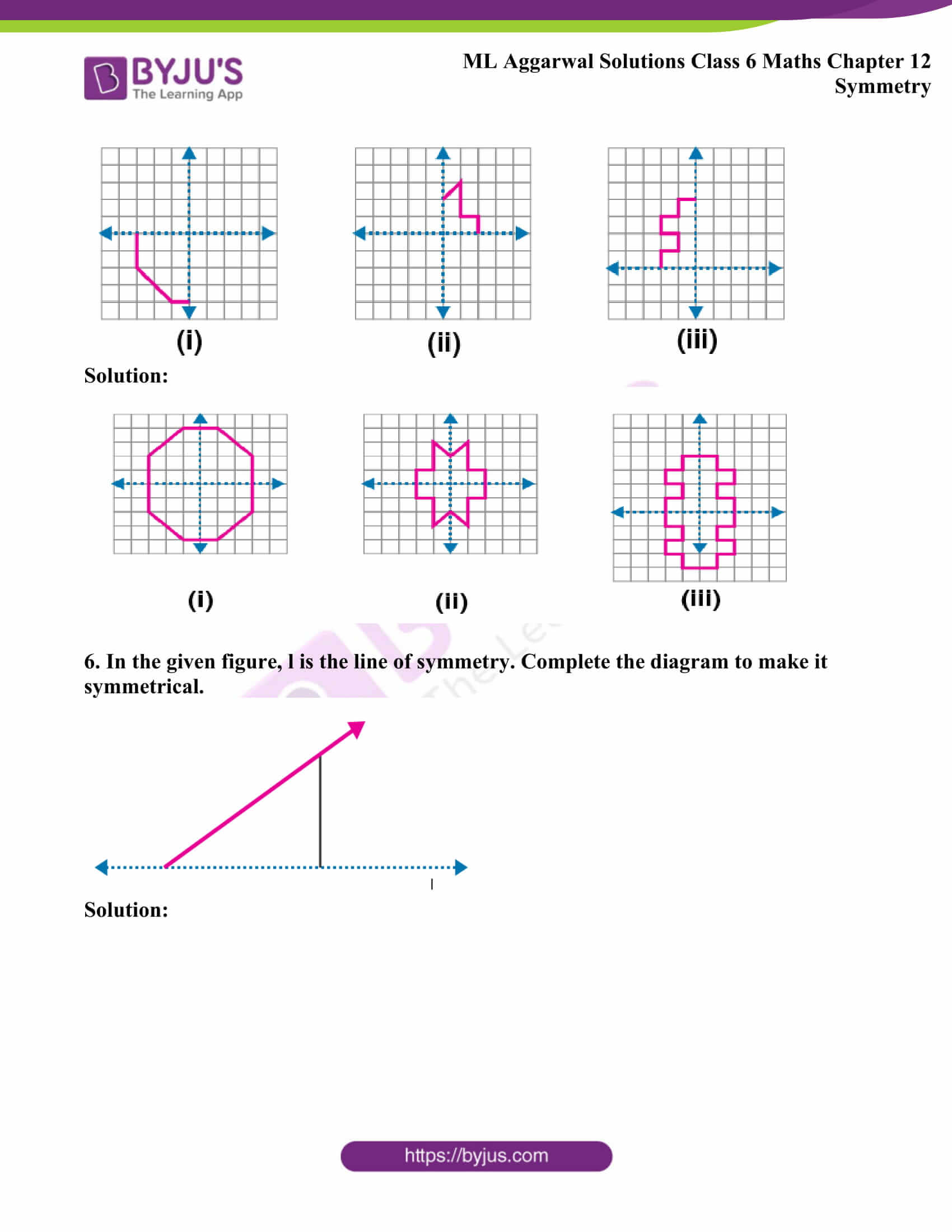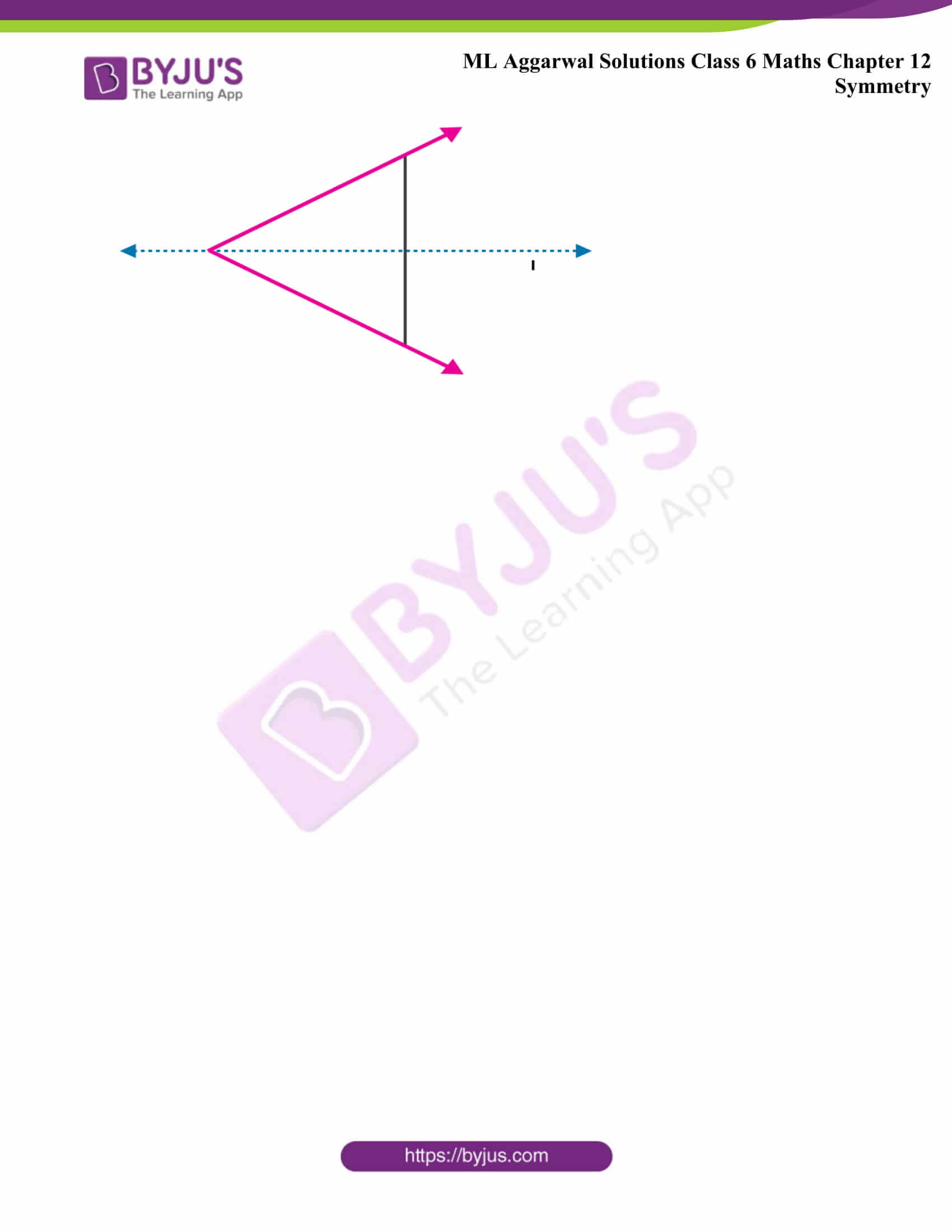## Access ML Aggarwal Solutions for Class 6 Maths Chapter 12 Symmetry

1. Draw the line (or lines) of symmetry, if any, of the following shapes and count their number.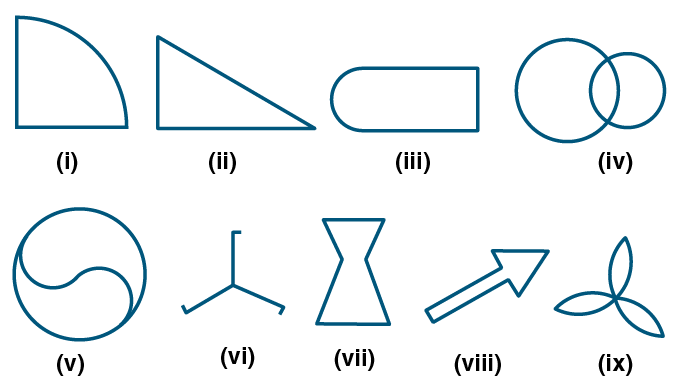Solution: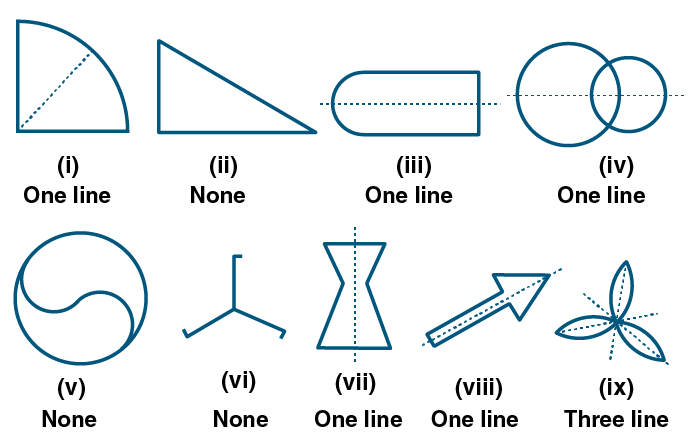2. Draw the line (or lines) of symmetry, if any, of the following pictures (of objects) and count their number: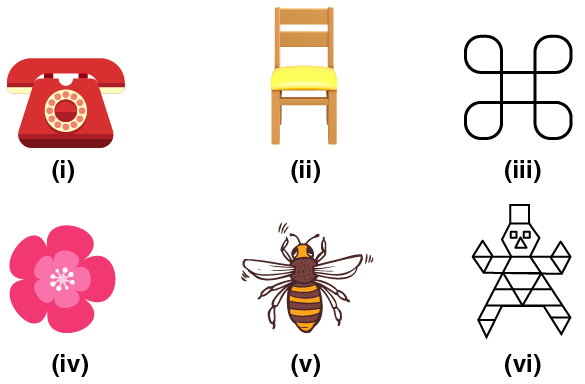Solution: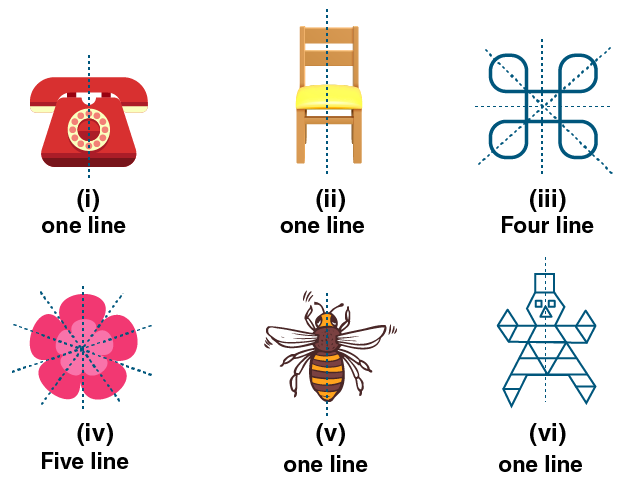3. Draw the line (or lines) of symmetry, if any, of the following road signs and count their number.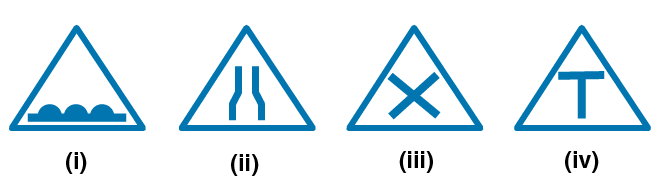Solution: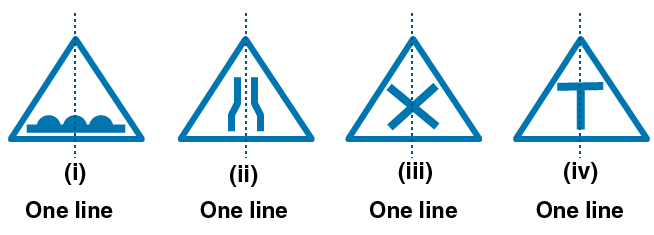4. Copy the following figures on a squared paper. Complete each of them such that the dotted line is the line of symmetry: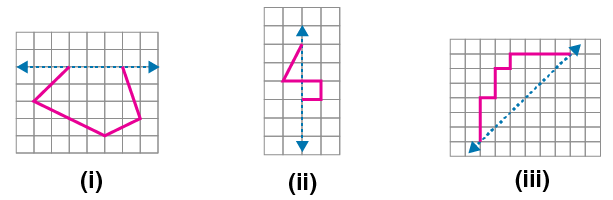Solution: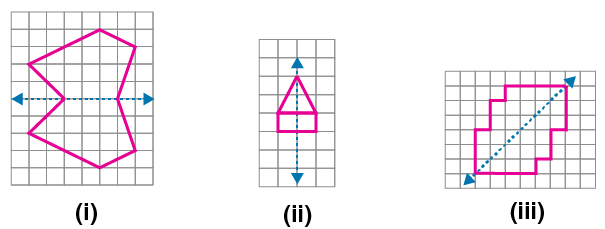5. Copy the following figures on a squared paper. Complete each of them such that the resultant figure has two dotted lines as the lines of symmetry: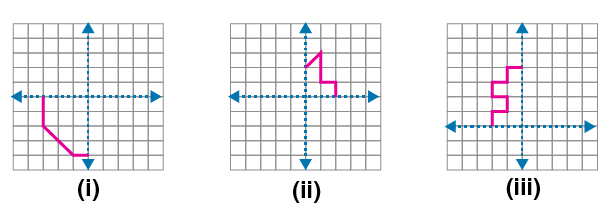Solution: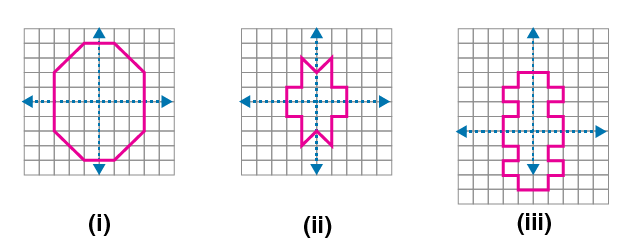6. In the given figure, l is the line of symmetry. Complete the diagram to make it symmetrical.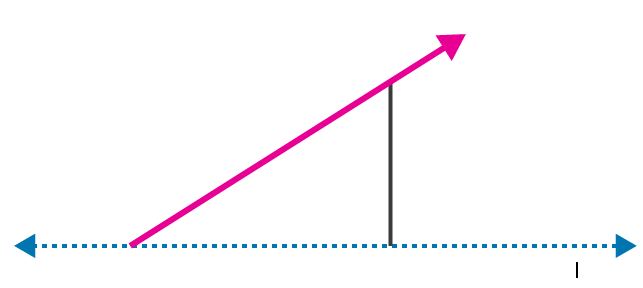Solution: